Courses

# Chopper -1

## 10 Questions MCQ Test Mock Test Series for Electrical Engineering (EE) GATE 2020 | Chopper -1

Description
This mock test of Chopper -1 for Electrical Engineering (EE) helps you for every Electrical Engineering (EE) entrance exam. This contains 10 Multiple Choice Questions for Electrical Engineering (EE) Chopper -1 (mcq) to study with solutions a complete question bank. The solved questions answers in this Chopper -1 quiz give you a good mix of easy questions and tough questions. Electrical Engineering (EE) students definitely take this Chopper -1 exercise for a better result in the exam. You can find other Chopper -1 extra questions, long questions & short questions for Electrical Engineering (EE) on EduRev as well by searching above.
QUESTION: 1

### Assertion (A): The principal of step-up chopper can be employed for the regenerative braking of dc motors. Reason (R) : The step-up chopper can be employed in regenerative braking only for increasing motor speeds

Solution:

The step-up chopper can be employed in regenerative braking both for increasing and decreasing motor speed provided duty cycle a is so adjusted that Vs/( 1 - a) exceeds the fixed source voiiage V0. Hence, reason is a false statement.

QUESTION: 2

### A dc chopper has a T0N of 1 ms and its frequency is 500 Hz. What will be its duty cycle?

Solution: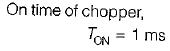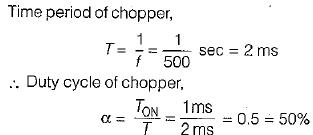QUESTION: 3

### An RLE load is operating in a chopper circuit from a 500 volts dc source as shown in figure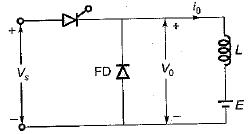For the load, L = 0.06H, R=0 Ω an duty cycle of 0.2, what is the chopping frequency to limit the amolitude of load current excursion to 10 A?

Solution: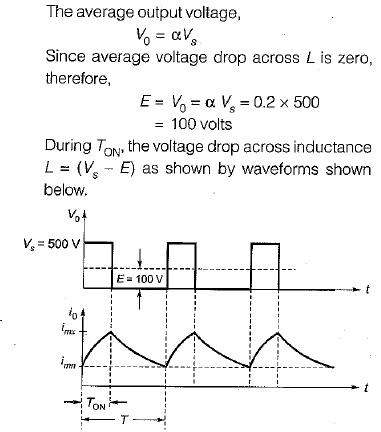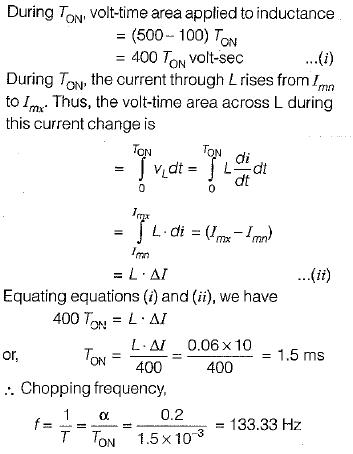QUESTION: 4

In a type-A chopper, the maximum value of ripple current is

Solution: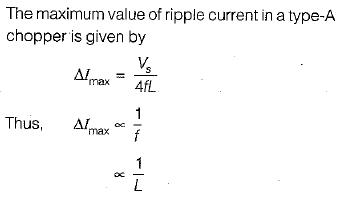QUESTION: 5

Match List-I (Thyristor chopper circuits) wtih List-ll (Characteristics) and select the correct answer using the codes given below the lists:
List-I
A. Current commutated chopper
B. Voltage commutated chopper

List-ll
1. Diode in antiparallel with main SCR
2. Used in high power circuits
3. No requirement of any commutating inductor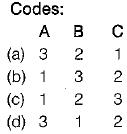Solution:

• In a current-commutated chopper a diode is connected in antiparallel with the main thyristor so that voltage drop across the diode reverse biases the main SCR.
• A voltage-commutated chopper is generally used in high-power circuits where load fluctuation is not very large.
• In a load-commutated chopper no commutating inductor is required that is normally costly, bulky and noisy.

QUESTION: 6

In dc choppers, per unit ripple is maximum when duty cycle α is

Solution:
QUESTION: 7

If T is the chopping period, Ton the on-time period of chopper and Toff the off-time period of the chopper, then consider the following statements associated with the methods of controlling the output voltage of a chopper:
1. In pulse-width modulation (PWM) scheme, T is kept constant while Ton is varied.
2. In variable-frequency modulation (VFM) scheme, T is varied while either of Ton or Toff is kept constant.
3. In PWM scheme, the output voltage can be varied between zero to source voltage.
4. The large Toff in VFM scheme may make the load current discontinuous
5. PWM scheme is better than VFM scheme.

Which of the statements given above are correct?

Solution:
QUESTION: 8

In dc choppers, the waveforms for input and output voltages are respectively

Solution:
QUESTION: 9

A class C chopper is operated from a 220 V battery. The load Is a dc motor with R = 0.1Ω, L= 10 mH and Eb= 100 V. The duty cycle of the chopper to achieve regenerative braking at the rated current of 10 Ampere would be equal to

Solution: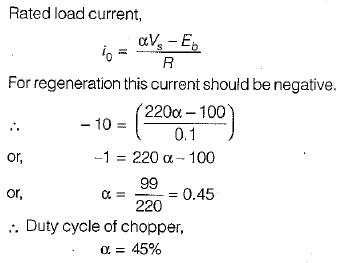QUESTION: 10

Assertion (A) : In dc choppers, it is essential to provide a separate commutation circuitry to commutate the main power SCR.
Reason ( R ) : A chopper is dc equivalent to an ac transformer having continuously variable turns ratio.

Solution:

Since the input supply to a dc chopper is fixed do voltage therefore, natural commutation is not possible in a dc chopper. Due to this reason a separate commutation circuit is required to commutate the main power SCR in a dc chopper. Reason is also a true statement but not the correct explanation of assertion.